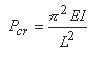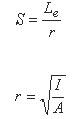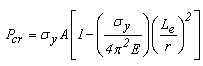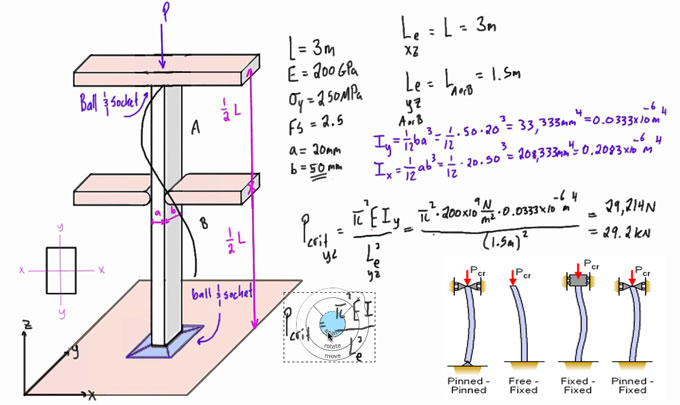# Calculation of buckling for pinned columnFor the ideal pinned column, the critical buckling load is computed with Euler's formula:Here:
E stands for Modulus of elasticity of the material
I stands for Minimum moment of inertia
L stands for Unsupported length of the column.

It should be remembered that irrespective of the end condition, the critical load is based not only on the material strength, but also on the flexural rigidity, EI. Buckling resistance is raised by enhancing the moment of inertia.

If a column buckles, it retains its deflected shape once the critical load is enforced. In most applications, the critical load is normally considered as the maximum load tolerable by the column. Theoretically, any buckling mode is feasible, but the column will ordinarily deflect into the first mode. A column will buckle when the load P attains a critical level, known as the critical load, Pcr.

For columns containing several types of support, Euler's formula may is useful when the distance L is substituted with the distance among the zero moment points. See "Effective Length Constant Table" below.

This length is known as the effective length Le and is demonstrated with the following equation:The slenderness ratio is a vital parameter in the classification of compression members, and is demonstrated with the following equation:Here:
r stands for Radius of gyration
I stands for Moment of inertia
A stands for Area cross section

If the slenderness ratio remains (greater than) critical slenderness ratio, then the column is considered as a long column and the Euler buckling formula will be as follow :-

If slenderness ratio remains less than the critical slenderness ratio, the column is considered as a short column.In short columns, failure may happen by compression devoid of major buckling and at stresses surpassing the proportional limit. For this condition, Johnson's formula will be used :For columns which collapse following the onset of inelastic behavior, the constant of proportionality should be utilized in spite of the modulus of elasticity (Engesser formula). The constant of proportionality, Et, refers to the slope of the stress-strain diagram beyond the proportional limit, known as the tangent modulus. Note within the linearly elastic range, E = Et.

To calculate column buckling, use the following online calculator engineersedge.com